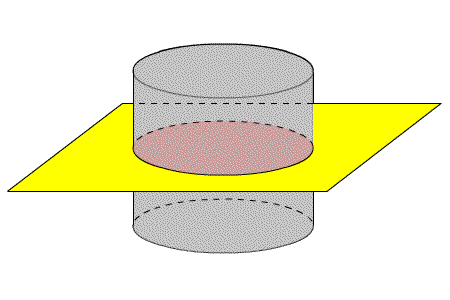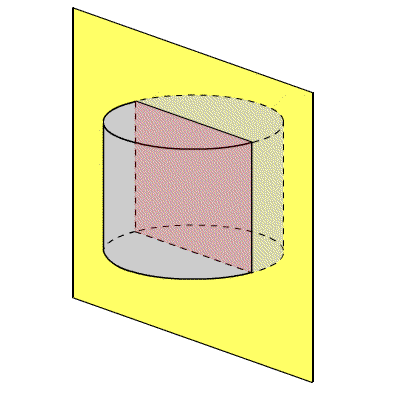# Cross Sections

A cross-section is a $2$ -dimensional “slice” of a $3$ -dimensional figure. Another way to think of it is finding the intersection of a $2$ -dimensional plane and a $3$ -dimensional figure.

For any given $3$ -dimensional figure, the cross-section depends on the orientation of the plane or “slice”. For example, consider a cylinder . Various cross sections might result in a circle or rectangle, as shown below, or any of several other shapes.